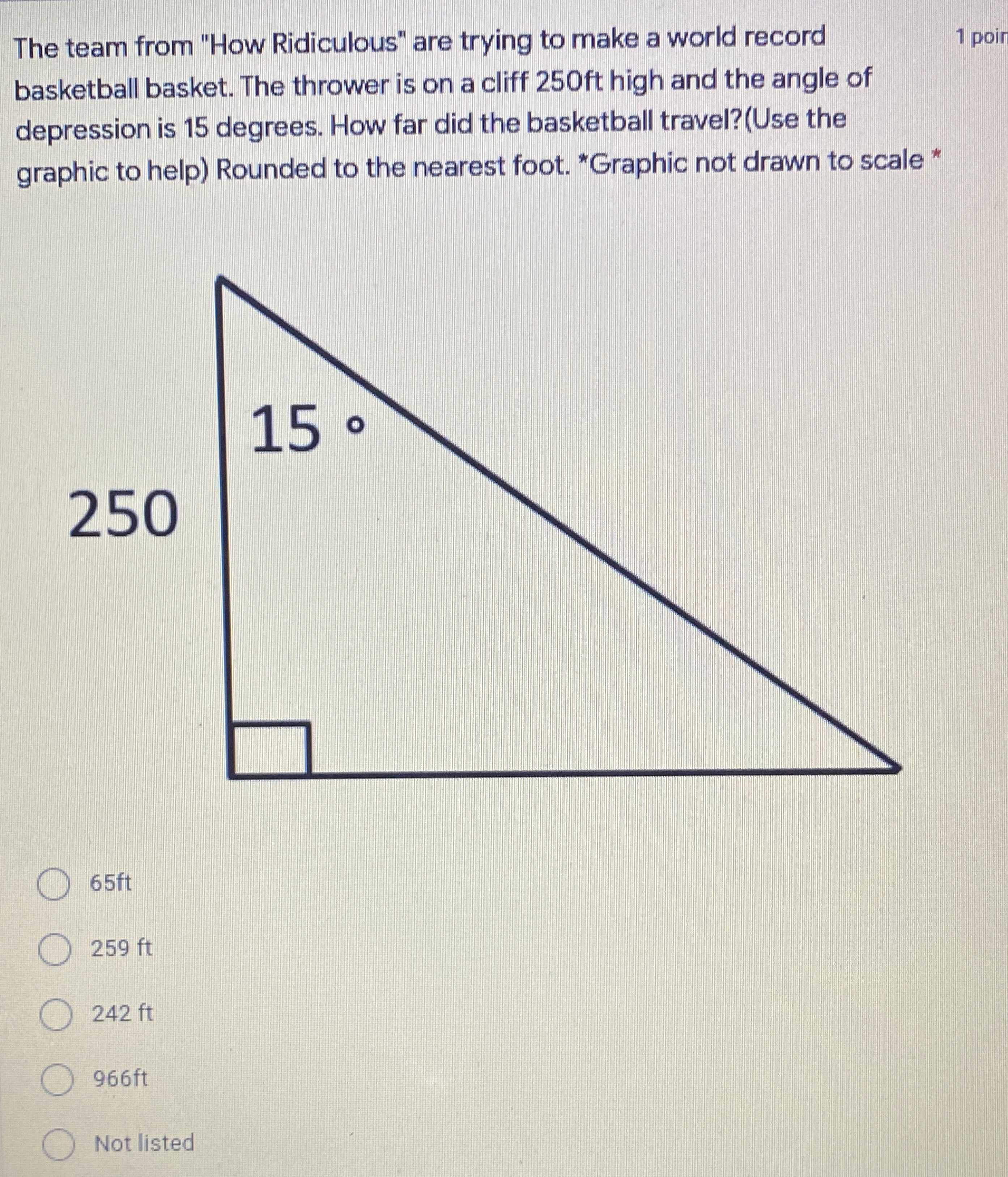### Still have math questions?

Trigonometry
QuestionThe team from "How Ridiculous" are trying to make a world record basketball basket. The thrower is on a cliff $$250 f t$$ high and the angle of depression is $$15$$ degrees. How far did the basketball travel?(Use the graphic to help) Rounded to the nearest foot. "Graphic not drawn to scale * $$65 ft$$

$$259 ft$$

$$242 ft$$

$$966 ft$$

Not listed

$$259 ft$$